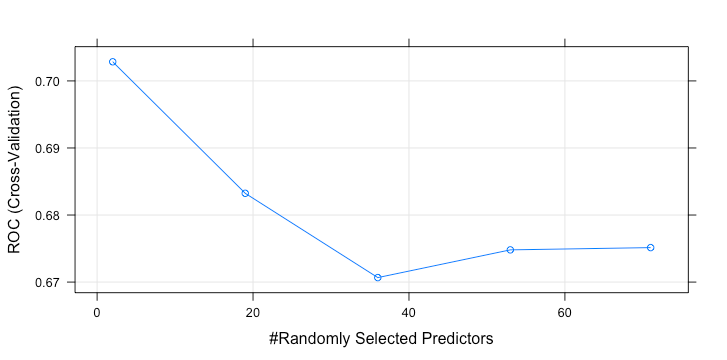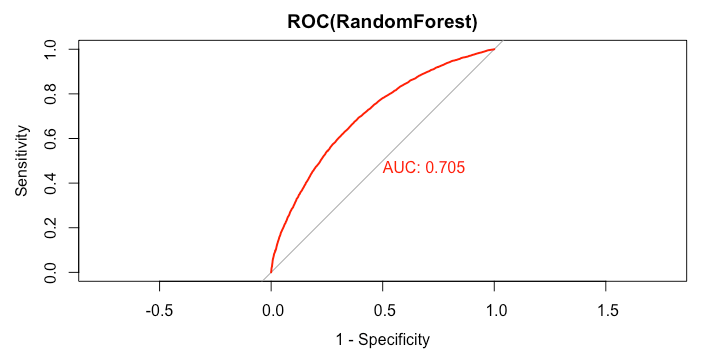# Random Forest Model in R

Now, we will tune RandomForest model. Like SVM, we tune parameter based on 5% downsampling data. The procedure is exactly the same as for SVM model. Below we have reproduced the code for Random Forest model.

set.seed(300)
samp = downSample(data_train[-getIndexsOfColumns(data_train, c( "loan_status") )],data_train$loan_status,yname="loan_status") #choose small data for tuning train_index_tuning = createDataPartition(samp$loan_status,p = 0.05,list=FALSE,times=1)
#choose small data for re-train
train_index_training = createDataPartition(samp$loan_status,p = 0.1,list=FALSE,times=1) rfGrid = expand.grid( .mtry = as.integer(seq(2,ncol(samp), (ncol(samp) - 2)/4)) ) #Install random forest package library(randomForest) rfTuned = train( samp[train_index_tuning,-getIndexsOfColumns(samp,"loan_status")], y = samp[train_index_tuning,"loan_status"], method = "rf", tuneGrid = rfGrid, metric = "ROC", trControl = ctrl, preProcess = NULL, ntree = 100 ) plot(rfTuned)> rfTuned Random Forest 1268 samples 70 predictor 2 classes: 'Default', 'Fully.Paid' No pre-processing Resampling: Cross-Validated (3 fold) Summary of sample sizes: 845, 845, 846 Resampling results across tuning parameters: mtry ROC Sens Spec 2 0.7028532 0.6909073 0.6199440 19 0.6832394 0.6451832 0.6088706 36 0.6706683 0.6231333 0.5820516 53 0.6748038 0.6263003 0.6026111 71 0.6751421 0.6609511 0.5962622 ROC was used to select the optimal model using the largest value. The final value used for the model was mtry = 2. >  The best parameter is mtry(number of predictors) = 2. Like SVM, we fit 10% of downsampling data with this value. rf_model = randomForest(loan_status ~ . ,data = samp[train_index_training,],mtry = 2,ntree=400) predict_loan_status_rf = predict(rf_model,data_test,"prob") predict_loan_status_rf = as.data.frame(predict_loan_status_rf)$Fully.Paid
rocCurve_rf = roc(response = data_test$loan_status, predictor = predict_loan_status_rf) auc_curve = auc(rocCurve_rf) plot(rocCurve_rf,legacy.axes = TRUE,print.auc = TRUE,col="red",main="ROC(RandomForest)")> rocCurve_rf Call: roc.default(response = data_test$loan_status, predictor = predict_loan_status_rf)

Data: predict_loan_status_rf in 5358 controls (data_test$loan_status Default) < 12602 cases (data_test$loan_status Fully.Paid).
Area under the curve: 0.705
>
predict_loan_status_label = ifelse(predict_loan_status_rf<0.5,"Default","Fully.Paid")
c = confusionMatrix(predict_loan_status_label,data_test$loan_status,positive="Fully.Paid") table_perf[3,] = c("RandomForest", round(auc_curve,3), as.numeric(round(c$overall["Accuracy"],3)),
as.numeric(round(c$byClass["Sensitivity"],3)), as.numeric(round(c$byClass["Specificity"],3)),
as.numeric(round(c\$overall["Kappa"],3))
)


The model’s performance is as follow:

> tail(table_perf,1)
model   auc accuracy sensitivity specificity kappa
3 RandomForest 0.705    0.657       0.666       0.635 0.268
>